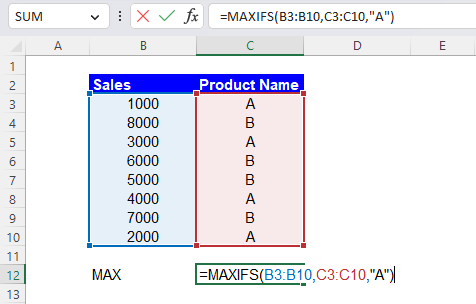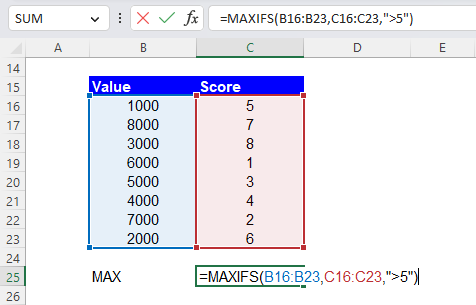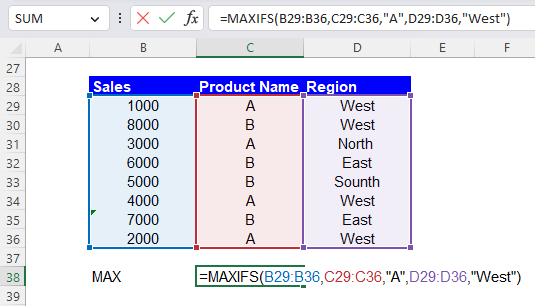Excel Formulas

# MAXIFS Function in Excel: Explained

In this article, you will learn how to use the MAXIFS formula in Excel.

## What is the MAXIFS function in Excel?

The MAXIFS function in Excel is a function that returns the maximum value from a range of cells that meet one or more criteria.

## When is the MAXIFS helpful in Excel?

The MAXIFS function can be helpful when you want to filter based on multiple criteria and find the maximum value that meets one or more conditions from a larger dataset. It can be helpful in different situations, such as:

1. Finding the highest sales figures for a specific product or region
2. Identifying the top-performing employee in a particular department or role based on his/her performance score
3. Determining the maximum temperature or precipitation on a specific day or month

The MAXIFS formula saves time and effort compared to manually filtering the data and finding the maximum value. It allows you to analyze data quickly and decide based on the results.

## How do you use the MAXIFS formula in Excel?

The MAXIFS function is generally used in the following way:

``=MAXIFS(max_range, criteria_range1, criteria1, [criteria_range2, criteria2], ...)``

“max_range”: This argument is the range of cells in which you want to find the maximum value.

criteria_range1: This is the range which the formula searches for “criteria1”.

criteria1: This is the criterion to be met in “criteria_range1”.

The subsequent arguments, “criteria_rangeX” and “criteriaX”, consist of pairs of criteria ranges and criteria. The function will return the maximum value from the max_range that meets all specified criteria.

Note: The size of the range or array for “max_range” and “criteria_rangeX” needs to be the same; otherwise the MAXIFS formula returns the #VALUE! error value. Also note that if you enter the text or date criteria manually in the function, don’t forget to enclose text with quotation marks.

Assume you have a list of sales figures in column B and a list of product names in column C. To find the highest sales figure for product "A", you would use the following formula:

``=MAXIFS(B3:B10, C3:C10, "A")``

In this example, the max_range is B3:B10, the criteria_range1 is C3:C10, and the criteria1 is "A". The formula returns 4,000, the largest value among the sales with Product Name of A, which are 1,000, 3,000, 4,000, and 2,000.How to use the MAXIFS function with a condition in Excel

Another example: Imagine you have a range of cells B16:B23 containing values and a range of cells C16:C23 containing criteria. You could use the following formula to find the maximum value in range B16:B23 where the corresponding value in C16:C23 is greater than 5.:

``=MAXIFS(B16:B23, C16:C23, ">5")``

This formula returns 8,000 because it is the maximum value among the values with scores over 5, which are 8,000, 3,000, and 2,000.How to use the MAXIFS function with a requirement  in Excel

You can also use multiple criteria like this example. Assume you have a list of sales figures in column B, a list of product names in column C, and a list of regions in column D. To find the highest sales figure for product "A" and region "West", you would use the following formula:

``=MAXIFS(B29:B36, C29:C36, "A", D29:D36, "West")``

In this example, the max_range is B29:B36, the criteria_range1 is C29:C36, the criteria1 is "A", the criteria_range2 is D29:D36, and the criteria2 is "West". The formula gives you 4,000, the maximum value among the sales with the Product Name of “A” in the “West” region, which are 1,000, 4,000, and 2,000.How to use the MAXIFS function with two criteria in Excel

## Why does the MAXIFS return zero in Excel?

The MAXIFS function in Excel may return zero if one or more of the following conditions are met:

1. The max_range is empty or selected wrongly: If the range of cells in which you want to find the maximum value is blank, the function will return zero.
2. The criteria do not match any cells: If the conditions do not match any cells in the criteria_range, the function will return zero. Ensure that the standards match the data in the criteria_range, and that the requirements are in quotes if they are text.
3. The max_range contains only non-numeric values: If the range of cells in which you want to find the maximum value has only non-numeric values, the function will return zero.
4. The max_range contains only zero or negative numbers: If the range of cells in which you want to find the maximum value contains only zero or negative numbers, the function will return zero.

Suppose you are experiencing a problem with the MAXIFS function returning zero or an error value. In that case, you should ensure that all arguments are correctly input and selected (in the case of cell references) and that the referred ranges contain the proper values to analyze.

## Analyze your live financial data in a snap in Google Sheets

Are you learning this formula to visualize financial data, build a financial model, or conduct financial analysis? In that case, LiveFlow may help you automate manual workflows, update numbers in real-time, and save time. You can access various financial templates on our website, from the simple Income Statement to Multi-Currency Consolidated Financial Statement. Are you interested in this product but are an Excel user? That’s not a problem at all. You can connect Google Sheets to Excel quickly.